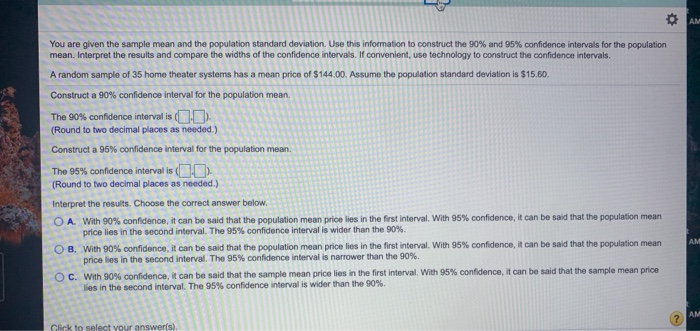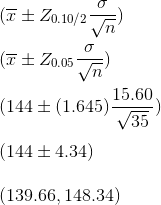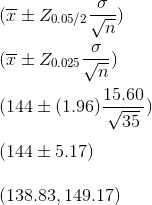Questiona)

We know that the 90% confidence interval for population mean is given by:b)

We know that the 95% confidence interval for population mean is given by:c)

So, with 90% confidence, it can be said that the population mean lies in 1st interval and with 95% confidence, it can be said that the population mean lies in 2nd interval. Also, 95% interval is wider than 90% interval.

Hence option A.

#### Earn Coins

Coins can be redeemed for fabulous gifts.

Similar Homework Help Questions